American Journal of Optics and Photonics
Volume 3, Issue 5, October 2015, Pages: 80-84

Electron-Hole and Photon Recombination Processes in Quantum Well Semiconductor Lasers

Barnbas Achakpa Ikyo

Dept.of Physics, Benue State University, Makurdi, Nigeria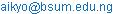(B. A. Ikyo)

Barnbas Achakpa Ikyo. Electron-Hole and Photon Recombination Processes in Quantum Well Semiconductor Lasers. American Journal of Optics and Photonics. Vol. 3, No. 5, 2015, pp. 80-84. doi: 10.11648/j.ajop.20150305.14

Abstract: The success obtained from semiconductor laser devices is dependent on the science of manipulating the electron-hole and the photon. While the most useful interaction in the laser is the recombination process that involves the electron and holes to produce a photon, other non-useful processes also occur and in some cases overshadow the preferred recombination process. In this review article, the physics behind radiative and non-radiative recombination processes and loss mechanisms dominant in quantum well semiconductor lasers is presented. The work concludes by suggesting possible solutions based on relevant published works

Keywords: Semiconductors,Lasers, Recombination, Quantum-Well

1. Introduction

When current is injected into a semiconductor laser, ideally one would expect that each injected (generated) electron-hole pair produces a photon. In reality there are other processes which may increase the threshold current (Ith) and deteriorate the device performance. The total current at threshold is a sum of the different terms depending on carrier concentration n, describing recombination via defects, radiative recombination, non-radiative Auger recombination and carrier leakage [1,2].

2. Photon-Interaction with Carriers in a Semiconductor

When photons of energy E interact with carriers in a semiconductor, the photon causes a transition of constituent carriers from one energy band to another leading to either a release of another photon or absorption of the interacting photon. Considering a semiconductor with filled electron states in the conduction band (hence forth referred to as CB) of energy E2 and empty states in the valence band (hence forth referred to as VB) of energy E1, three possible ways have been identified by which electrons in the two bands can interact. These interactions are; absorption, spontaneous emission and stimulated emission and are schematically presented in figure 1. The relationship between the band energies and the photon energy is given in equation 1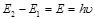(1)

Where E, is the photon (transition energy), h is plank’s constant and v is the frequency of photon.

2.1. Absorption

In an absorption process schematically shown in figure 1(a), the energy of an incident photon is absorbed by an electron in the VB of energy E1, the electron is excited to the CB of energy E2. The pre-condition for this process is that, the incident photon energy E12 will satisfy the condition of equation 1. The probability of absorption is proportional to the density of electrons in the VB, the density of un-occupied states in the CB and the photon density. The rate of absorption for such a system is defined by equations 2.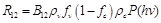(2)

Where,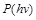is the density of incident photons and B12 is the Einstein’s absorption rate coefficient.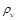and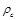are the densities of states for the VB and CB respectively. fc and fv are the probability distribution of the electron and holes described by the Fermi-Dirac distribution function for electrons in the CB and empty state in the VB.

2.2. Spontaneous Emission

Spontaneous emission occurs when an electron loses energy and transfers from the CB to VB emitting a photon of energy E21 satisfying the condition, in the process illustrated in figure 1(b). The cause of spontaneous emission is attributed to the interaction of the electron with a virtual photon. The rate of decrease of the electron number density of electrons in the CB (spontaneous emission rate) during spontaneous emission process is given by equation 3. The probability of spontaneous emission is proportional to the density of electrons in the CB and empty states in the VB.(3)

Where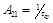is the spontaneous emission lifetime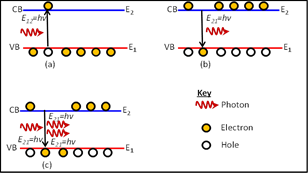Figure 1. Two state energy level model showing (a) absorption, (b) spontaneous emission and (c) stimulated emission.

2.3. Stimulated Emission

Stimulated emission occurs when an incident photon causes an electron in the CB to transfer to the VB, releasing a photon. The energy of the incident photon must satisfy equation 1. The incident photon is not absorbed in this case but it leaves the system with the emitted photon as pure monochromatic light, it also has equivalent energy, frequency and phase to that of the incident photon. The rate of stimulated emission is proportional to the density of electrons in the CB, the density of empty states in the VB and the photon density,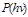and can be expressed by equation 4.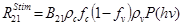(4)

It has been shown that, B12=B21=B  and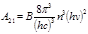.

3. Recombination Processes in Semiconductor Lasers

When current is injected into a semiconductor laser, ideally one would expect that each injected (generated) electron-hole pair produces a photon. In reality there are other processes which may increase the threshold current (Ith) and deteriorate the device performance. The total current at threshold is a sum of the different terms depending on carrier concentration n, describing recombination via defects, radiative recombination, non-radiative Auger recombination and carrier leakage [1,2] given by equation 5.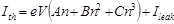(5)

Where, e is the electron charge, V is the volume of the active region A, B and C are recombination coefficients of the monomolecular (recombination at defects), radiative and Auger recombination, respectively.

In a direct band semiconductor laser, radiative recombination is a process where an electron in the conduction band recombines with a hole in the valence band releasing a photon as illustrated in figure 2(a). This process may either be spontaneous or stimulated. Radiative recombination due to spontaneous emission may be expressed as ;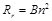and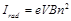Here, B is the radiative recombination coefficient. It is worth mentioning that the measured pure integrated spontaneous emission Lspon is directly proportional to the radiative current Irad at threshold. This is the basis for the experiment used to extract the various current paths of the diode laser threshold current; the method is also used in this work.

When a semiconductor laser is injected with current, the desired recombination in the active region is an electron-hole pair that emits a photon. But in reality, there are other processes which cause carrier loss thereby contributing to the threshold to the detriment of the device performance. Mechanisms such as recombination at defects, Auger recombination and leakage current are the main non-radiative processes [1,4].

3.3. Recombination at Defects

Defects arise from the deviations in the crystalline structure of the semiconductor material. Defects are usually created during epitaxial growth; an atomic site can be substituted by an impurity, or change position leaving a vacant site which would normally be occupied, or it is sometimes by an atom occupying a supposedly empty site. These defects states (electrons and holes) within a diffusion length may recombine non- radiatively [5,6]. The rate of defect related recombination is expressed as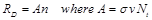(6)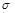, is the capture cross section of the defects, Nt is the density of defects, and v is the velocity of electrons and holes. The current due to the non-radiative (monomolecular) recombination is referred to as the defects current (IDefects) and is the first term of equation 7 given as;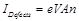(7)

3.4. Auger Recombination

In non-radiative Auger recombination, the energy of the recombining electron and hole is gained by another electron (hole) which is excited to a higher (lower) energy level in the conduction (valence) band . To achieve thermal equilibrium, the excited carrier relaxes through lattice vibration and emits a phonon. The Auger carrier lifetime is given by the equation 8.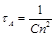(8)

From this relation, C can be evaluated experimentally by measuring carrier lifetimes and the carrier density. C is the Auger recombination coefficient it is in itself not dependent on carrier density but is thermally activated and in bulk materials and can be expressed by equation 9. Where C0 is independent of temperature and Ea is the activation energy.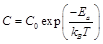(9)

The Current due to the Auger recombination is referred to as Auger current and is given as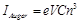(10)

Auger recombination is band gap sensitive and increases as the band gap of the semiconductor decreases and as a result it is a major problem in mid infrared devices . This is because as the band gap decreases, the activation energy and effective mass of carriers reduces thereby increasing the probability of the Auger process as shown in figure 2. The percentage contribution of non-radiative Auger recombination to the threshold current of diode lasers can be estimated experimentally by varying the band gap of the devices using temperature and hydrostatic pressure. These two techniques have been employed in this work and a detailed explanation of the procedure will be given in chapter three of this thesis. There are many types of Auger recombination however only band-to-band Auger processes are discussed in detail.

Discussions here will be limited to the direct band gap semiconductor material and the parabolic band model will be used for illustrations. The band-to-band Auger process is one in which the momentum is conserved in an electron-hole transition and is not assisted by phonons. It is categorised into three based on the bands in which the Auger carriers reside and or are excited to.

In a conduction-hole Conduction-Conduction (CHCC) Auger process, an electron makes a transition from conduction to valence without emitting a photon; instead the energy excites another conduction band electron to a higher energy state. The excited electron later loses energy to optical phonons and the system attains thermal equilibrium . The illustration for this Auger process is shown in figure 2 (b).

Another form of direct band-to-band Auger is the Conduction-Heavy Hole- Spin-split off band-Heavy-hole Auger recombination (CHSH) shown in figure 2(c). In this Auger process, an electron from the spin-split off band is excited to the heavy hole band. If the electron-hole process leads to a transition from a light-hole band to the heavy-hole, the process is called the conduction-hole-light-hole-heavy-hole (CHLH) Auger process illustrated in figure 2 (d).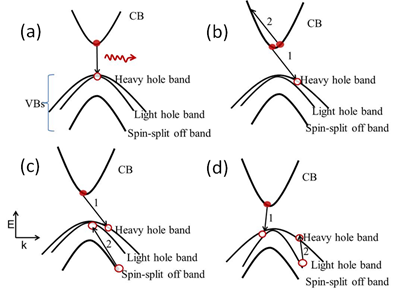Figure 2. Electron-hole recombination in (a) radiative process, (b) conduction-heavy hole-conduction-conduction Auger process, (c) conduction-heavy hole-spin-split off–hole Auger process and (d) the conduction-hole light hole-heavy hole Auger process. In (b), (c) and (d), a photon is not emitted; instead the transition energy is gained by a third carrier.

3.5. Carrier Leakage

Carrier leakage is a loss process where electrons or holes in a well layer escape over a barrier into the confining layer. Figure 3 show the schematic diagram of carrier leakage in a QW. Carrier leakage depends on the barrier height and the electron and hole mobility, due to the larger effective mass of holes, they are less mobile thus electron leakage is significantly higher for a given band offset [9,10]. Carrier leakage increases as temperature increases since carriers gain enough thermal energy to escape from the quantum well.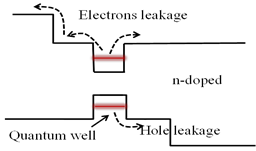Figure 3. Schematic diagram showing carrier leakage from a quantum well into the barrier and cladding layers.

The leaked carriers do not participate in the lasing process rather, they may recombine in the cladding layer non-radiatively (causing heating) thus increasing Ith. This loss is a general problem to diode lasers, increasing the band offset of quantum well lasers reduces carrier leakage.

3.6. Inter-Valence Band Absorption (IVBA)

Inter-valence band absorption (IVBA) is an optical loss process in which photo-absorption excites an electron from a lower energy level to a higher one within the VB of a semiconductor laser. IVBA could involve the transition of electrons from the light-hole band to the heavy-hole band, from the spin-split band to the light-hole band or from the spin-split off band to the heavy-hole band. Due to the high density of states and hole occupancy in the heavy hole band and the more likely resonance of ΔSO with the bandgap energy, the IVBA involving these two bands is more important . The transition energies of the light hole-heavy hole process are too small or too far out of k-space and would normally be out of resonance. Also, the spin split-off-light-hole process may as well be neglected in high ΔSO devices since it requires carriers at high k. Calculated absorption coefficients for ternary materials are found to increase with increasing temperature. This implies that, IVBA is a temperature dependent optical loss process and it will easily couple with Auger recombination to degrade device performance at ambient temperatures. The explanation to this temperature dependence is that, as temperatures increase the hole population changes as carriers are distributed deep down the heavy-hole band and further out in k. This increases the probability of IVBA occurring.

4. Conclusion

Ideally, each electron injected into a laser should recombine to produce a photon. This would mean that the quantum efficiency of the laser will be 100% . This does not occur due to the reasons evaluated above. Non-radiative recombination is also the reason for high threshold current in most semiconductor devices. This affects the low gap-short wavelength lasers since the probability of Auger recombination occurring is higher is such devices. These effects can be mitigated through growth of strained layers within quantum well materials. carrier leakage is another path through which injected electron are lost without taking part in the lasing process, leaked carriers can recombine out the well or loose energy through phonon interaction . This can be controlled through efficient engineering of the band offset making use of appropriate barriers.

While the Auger and carrier leakage affect the injected electron directly, IVBA prevails from the supplies from emitted photons. When the energy of the photon is resonance with sub-bands in the VB including ΔSO, photons may be absorbed and not allowed to leave the laser cavity thereby reducing the overall laser efficiency. This can be measured in form of external differential efficiency.

There is therefore critical need to understand the physics behind these processes and ways to measure each of them in semiconductor lasers.

Acknowledgements

I would like to thank the Nigerian Government that sponsored my PhD through PTDF. I also appreciate the teaching and discussions on semiconductor lasers by Prof. Stephen Sweeney and Alf Adams at the University of Surrey.

References

1. M. Nathan, W. Dumke, G. Burns, F. Dill, and G. Lasher, "- stimulated emission of radiation from GaAs p?n JUNCTIONS," vol. - 1, 1962.
2. N. Holonyak and S. Bevacqua, "- coherent (visible) light emission from Ga(As1-xPx) junctions," vol. - 1, 1962
3. K. O'Brien, S. J. Sweeney, A. R. Adams, B. N. Murdin, A. Salhi, Y. Rouillard, and A. Joullie, "Recombination processes in midinfrared InGaAsSb diode lasers emitting at 2.37 mu m," Applied Physics Letters, vol. 89, Jul 31 2006..
4. Z. Yin and X. Tang, "A review of energy bandgap engineering in III–V semiconductor alloys for mid-infrared laser applications," Solid-State Electronics, vol. 51, pp. 6-15, 2007..
5. W. W. Bewley, C. L. Felix, I. Vurgaftman, D. W. Stokes, J. R. Meyer, H. Lee, and R. U. Martinelli, "Optical-pumping injection cavity (OPIC) Mid-IR "W" lasers with high efficiency and low loss," Ieee Photonics Technology Letters, vol. 12, pp. 477-479, May 2000.
6. R. Q. Yang, J. L. Bradshaw, J. D. Bruno, J. T. Pham, and D. E. Wortman, "Mid-infrared type-II interband cascade lasers," Ieee Journal of Quantum Electronics, vol. 38, pp. 559-568, Jun 2002.
7. K. O'Brien, S. J. Sweeney, A. R. Adams, S. R. Jin, C. N. Ahmad, B. N. Murdin, A. Salhi, Y. Rouillard, and A. Joullie, "Carrier recombination mechanisms in mid-infrared GaInAsSb quantum well lasers," Physica Status Solidi B-Basic Solid State Physics, vol. 244, pp. 203-207, Jan 2007.
8. W. W. B. I. Vurgaftman., C.L. Canedy, C.S. Kim, M.m. Kim, C.D. Mmerritt, J. Abell, J.R. Lindle & J.R. Mmeyer, "Rebalancing of internally generated carriers for mid-infrared interband cascade lasers with very low power consumption," Nature communication, vol. 10, 2011.
9. T. Chen, S. Margalit, U. Koren, K. Yu, L. Chiu, A. Hasson, and A. Yariv, "- Direct measurement of the carrier leakage in an InGaAsP/InP laser," vol. - 42, 1983.
10. G. Belenky, Kazarinov, R., Lopata, J., Luryi, S., Tanbun-Elk,. T., Garbinski, P., "Direct measurement of Carrier Out of Active Region in InGaAsP/InP Laser Heterostructures," IEEE Transaction on Electron Devices, vol. 42, p. 4, 1995.
11. Numai, T., Fundamentals of semiconductor lasers. 2015: Springer
12. She, C., et al., Low-threshold stimulated emission using colloidal quantum wells. Nano letters, 2014. 14(5): p. 2772-2777

 Contents 1. 2. 2.1. 2.2. 2.3. 3. 3.1. 3.2. 3.3. 3.4. 3.5. 3.6. 4.
Article ToolsAbstractPDF(496K)